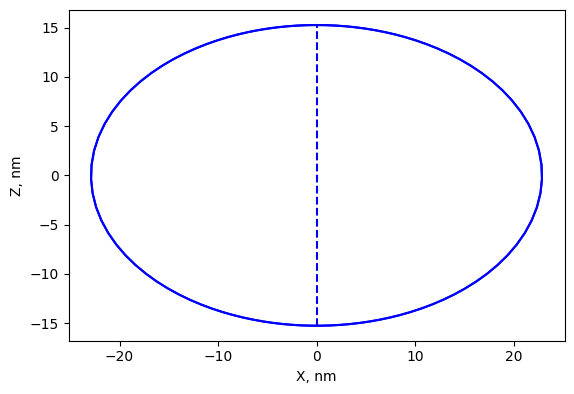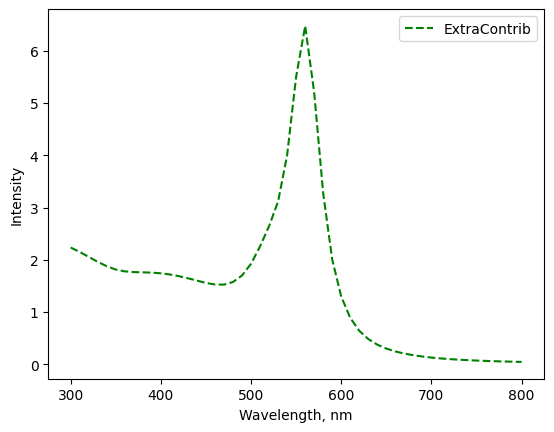# Non-spherical particles¶

T-matrix formulation allows to perform computational efficient calculations for single axially-symmtric objects. Currently supported only spheroid shape (rotational ellipsoid) using external library ScatterPy <https://github.com/TCvanLeth/ScatterPy>. The details can be found in [Mishchenko1998].

## Example¶

Calculation of extinction for oblate spheroid with aspect ratio of $$\alpha=a/c=1.5$$ . The size is specified by diamter $$a_{eff}$$ of equivalent-volume sphere.

The spheroid size can be derived as

$\begin{split}a = a_{eff} \alpha^{1/3} \\ c = a / \alpha\end{split}$
from mstm_studio.alloy_AuAg import AlloyAuAg
from mstm_studio.contrib_spheroid import SpheroidSP
import numpy as np

wls = np.linspace(300, 800, 51)  # range for calculation, in nm
SIZE = 20  # nm, particle diameter
ASPECT = 1.5  # a / c = horiz. axis / rot. axis

sph = SpheroidSP(wavelengths=wls)  # create object
sph.set_material(AlloyAuAg(x_Au=1), 1.5)  # particle and matrix refr. ind.fig, axs = sph.plot_shape([1, SIZE, ASPECT])
fig.savefig('spheroid_shape.png', bbox_inches='tight')

ext_sph = sph.calculate([1, SIZE, ASPECT])
fig, axs = sph.plot([1, SIZE, ASPECT])  # scale, diameter, aspect
fig.savefig('spheroid_ext.png', bbox_inches='tight')## Classes¶

Contributions to optical extinction spectra from axial-symmetric particles. Currently, spheroids.

class mstm_studio.contrib_spheroid.SpheroidSP(wavelengths=[], name='ExtraContrib')[source]

Extinction from spheroid calculated in T-matrix approach using external library ScatterPy <https://github.com/TCvanLeth/ScatterPy>

Parameters:

wavelengths: list or numpy array
wavelengths in nm
name: string
optional label
calculate(values)[source]

Parameters:

values: list of parameters scale, size and aspect
Scale is an arbitrary multiplier. Size parameter is the radius of equivelent-volume sphere. The aspect ratio is “the ratio of horizontal to rotational axes” according to scatterpy/shapes.py

Return:

extinction efficiency array for spheroid particle
plot_shape(values, fig=None, axs=None)[source]

Plot shape profile. Spatial shape is achieved by rotation over vertical axis.

Parameters:

values: list of control parameters
scale, size and aspect

fig: matplotlib figure

axs: matplotlib axes

Return:

filled/created fig and axs objects
 [Mishchenko1998] Mishchenko, L. Travis, “Capabilities and limitations of a current FORTRAN implementation of the T-matrix method for randomly oriented, rotationally symmetric scatterers ” (1998) 309